Physical Chemistry : Isothermic, Isobaric, and Adiabatic Processes

Example Questions

Example Question #1 : Isothermic, Isobaric, And Adiabatic Processes

Which of the following is not true for an isothermal process?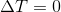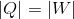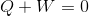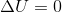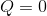Explanation:

For an isothermal process there is no change in temperature, therefore, the temperature is a constant. An ideal gas has an internal energy,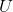, that is proportional to temperature, so if the temperature is does not change the internal energy does not change. Hence the name isothermal (iso means the same and thermal means temperature).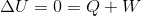If you rearrange the equation, you will find that: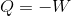A simple explanation of this is that all the heat applied to the system is used to do the work. A piston is a good example for this phenomenon. This happens when every bit of energy applied or removed from a piston as work is done so slowly that all the heat has enough time to conduct into or out of the system and maintain a constant temperature.

Example Question #2 : Isothermic, Isobaric, And Adiabatic Processes

A sample of an ideal gas is initially at a volume of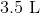. The gas expands to a volume of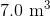when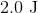of heat is applied to the system against a constant external pressure of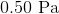. Calculate the change in internal energy for this gas.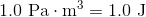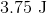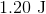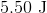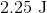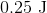Explanation:

The expression for the relationship between heat (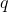) and work (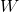) is change in internal energy (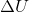):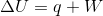The work () done by a system is: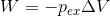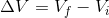with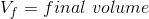and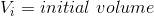Plugging work () into the internal energy equation gives: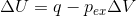Plugging the values given into the internal energy equation gives: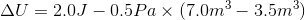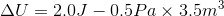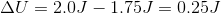Example Question #3 : Isothermic, Isobaric, And Adiabatic Processes

At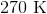, a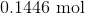sample of argon gas expands reversibly from a confined space of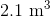to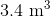. Calculate the work done.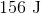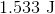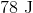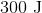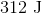Explanation:

The process as described by the question is an isothermal process. An isothermal process has a constant temperature, therefore, there is no change in temperature. For an isothermal reversible process, the work done by the system is: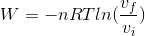Plugging the values given into the equation gives: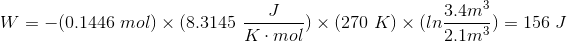Example Question #4 : Isothermic, Isobaric, And Adiabatic Processes

A sample of an ideal gas is initially at a volume of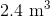. The gas expands to a volume of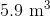when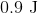of heat is applied to the system against a constant external pressure of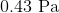. Calculate the change in internal energy for this gas.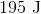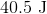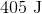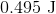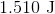Explanation:

The expression for the relationship between heat () and work () is change in internal energy ():The work () done by a system is: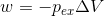withandPlugging work () into the internal energy equation gives:Plugging the values given into the internal energy equation gives: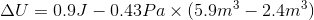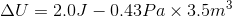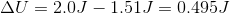Example Question #5 : Isothermic, Isobaric, And Adiabatic Processes

At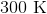, a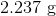sample of argon gas (atomic mass=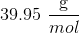) expands reversibly from a confined space of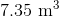to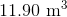. Calculate the work done.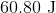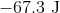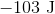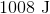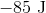Explanation:

The process as described by the question is an isothermal process. An isothermal process has a constant temperature, therefore, there no change in temperature. For an isothermal reversible process, the work done by the system is:Convert the grams to moles of argon: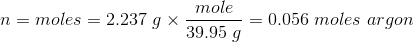Plugging the values given into the equation gives: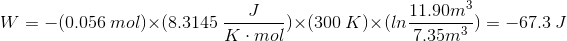Example Question #6 : Isothermic, Isobaric, And Adiabatic Processes

Which of the following processes are considered an isothermic reaction?

I. Condensation

II. Evaporation

III. Sublimation

I only

II only

I, II and III

I and II

I, II and III

Explanation:

Isothermic reactions are characterized as reactions that occur in constant temperature. Recall that phase changes (such as condensation, evaporation, etc.) occur at a constant temperature (melting point, boiling point,e etc.). Consider the following example. Water boils at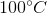; this means that when liquid water is placed at its boiling point, it will convert rapidly to gas (water vapor). The reaction will happen at a constant temperature (at) and, therefore, will be an isothermic process. This is true for all phase change reactions. Condensation is conversion of solid to liquid, evaporation is conversion of liquid to gas, and sublimation is conversion of solid to gas (or gas to solid).

Example Question #1 : Isothermic, Isobaric, And Adiabatic Processes

Melting of a solid to a liquid is an example of what type of reaction?

Isovolemic

Isothermic

More than one of these are true

Isobaric

Isothermic reactions occur in constant temperature, isovolemic reactions occur in constant volume and isobaric reactions occur in constant pressure. During phase changes, like melting, the temperature stays constant (at the melting point). The pressure will also likely remain constant because most reactions are carried out at atmospheric pressure (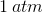); therefore, melting an ice cube is an isothermic and an isobaric reaction.